# Using External Attenuation to Improve Test Accuracy

Publish Date: Nov 22, 2017 | 4 Ratings | 3.75 out of 5 | Print | 6 Customer Reviews | Submit your review

## Overview

Use of external attenuation with a RF signal generators is a common practice used for several purpose. In this document, learn how external attenuation can be used to improve amplitude accuracy and reduce the noise density of an RF test stimulus.

### 1. Introduction

If you’ve watched television within the last two years, you’ve probably cringed as you heard the Cingular guy ask: “Can you hear me now?” for what seems like the thousandth time.  The marketing message behind this campaign claims that Cingular has better cell phone reception than many of their competitors.  While I certainly can’t complain about my 3G coverage in Austin, the marketing message doesn’t quite tell the whole story.  More specifically, what they don’t tell you is that your coverage in remote locations is as much a function your phone’s sensitivity as it is the actual network coverage.

Cellular transceivers aren’t the only devices for which sensitivity is important.  If you’re familiar with GPS (global positioning system), you might know that it’s one of the lowest power signals among all commercial communications system.  With a typical signal strength of -140 dBm, C/A (course acquisition) GPS receivers in the L2 (1.575 GHz) band are extremely sensitive to noise and interference.  Compared to a typical cellular base station (often around -70 dBm with a 1 dBi dipole antenna), GPS is an extremely weak signal.  In fact, GSM (cellular) signals are approximately 1,000,000 times the strength of GPS satellites on the earth’s surface.

As one might expect, characterizing highly sensitive receivers at low power levels requires careful attention to several specific details.  In this document, we will address how some challenges can be solved by “padding” the output of the test instrument with an external RF attenuator.  Most commonly, this method is employed to: 1) improve amplitude accuracy and 2) reduce the output noise floor of the test stimulus.  In this paper, we explain attenuator theory of operation and their use in RF instruments.  In addition, we will explain how external attenuators can be used to improve instrument performance in low-power test cases.

### 2. Attenuator Theory of Operation

To start with, let’s evaluate basic characteristics of a passive attenuator.  As the name suggests, this component produces an output signal that is lower in power than its input.  Most attenuators that you buy off the shelf come with fixed attenuation at levels such as 3 dB, 6 dB, 10 dB, 20 dB, or even more.  Mathematically, we can describe attenuation with a simple equation, shown below.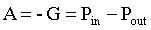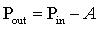Equations 1 and 2.  Relationship between power and attenuation.

All terms in both equations are expressed in terms of dB.  Based on equations 1 and 2, it is clear that if we have a signal at -40 dBm, a 20 dB attenuator will reduce the power level to -60 dBm.

In order to understand the use and limitations of external attenuators in practical applications, it is important to discuss the RF attenuation available on today’s signal generators.  To start with, let’s first evaluate where attenuation might be present on a supherheterodyne vector signal generator, illustrated in the figure below: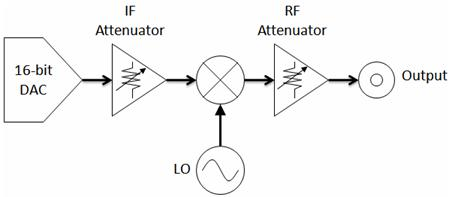Figure 1. Diagram of a superheterodyne vector signal generator.

While the diagram in figure1 is over-simplified (we’ve stripped filters, amplifiers, etc.), we can see that attenuation is applied at either the IF or RF stage.  In this diagram, a digital to analog converter (in this case 16-bit) is used to generate an IF signal.  Note that a small signal loss will also occur at the mixer, but we will assume the mixer loss is 0 dB for the sake of argument in this paper.  On the whole, programmable IF and RF attenuation are the most prominent ways to reduce signal power.

For example, suppose you wanted to generate CW tone at 1 GHz at a power level of -60 dBm.  In this scenario, an IF signal is generated with a 16-bit DAC at a voltage level of approximately 1 Vptp.  In order to understand the level of attenuation that must be applied to attenuate this signal to -60 dBm, let’s first understand how 1 Vptp might translate to a power level in dBm with the following: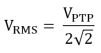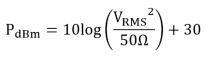Equations 3 and 4.  Power as a function of peak-to-peak and RMS voltage.

Using the equations above, we determine that a 1 Vptp signal has a power level of approximately +4 dBm.  Thus, assuming there is zero path loss in the RF signal chain, the generator must apply 64 dB of attenuation to produce the desired power level of -60 dBm.

Highly sensitive receivers, however, often require a test stimulus with power levels well below the maximum attenuation IF or RF attenuation available on the instrument.  In this scenario, digital attenuation must also be applied before digital-to-analog converter to reduce the IF power.  By applying digital attenuation, the full-scale range (and also full dynamic range) of the DAC is no longer used due to bit quantization.  However, the “unused” part of the dynamic range is the portion that would have been otherwise buried in the noise floor of the instrument.  In fact, external attenuation is required to extend dynamic range below the noise floor of the instrument.

We can prove this theoretically by first examining the relationship between dynamic range and bit resolution of a typical digital-to-analog converter (DAC).  Generally, a digital-to-analog converter produces 6.02 dB of dynamic range for each bit of resolution.  More specifically, we can represent the dynamic range as a function of the number of bits, ‘n’, in the following equation: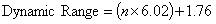Equation 5.  Dynamic range as a function of number of bits

Thus, a 16-bit DAC will have a theoretical dynamic range of ((16 x 6.02) + 1.76) = 98.08 dB.  Of course, the theoretical value is based purely on bit quantization and does not account for non-linear signal products which cause spurs, harmonics, and other types of distortion.  For the sake of argument, lets assume that our distortion products are 10 dB, resulting in a usable dynamic range, SFDR (spurious free dynamic range), of 88 dB (98 – 10 dB).

Based on our calculation of dynamic range for a 16-bit DAC, it is easy to see why digital IF attenuation is often used at lower power levels.  As an example, suppose you wanted to generate a signal at -120 dBm.  If an instrument produces an average noise density of -160 dBm/Hz, only 40 dB of SFDR is required to generate this signal.  Thus, if our DAC has 88 dB of SFDR, up to 48 dB (88 - 40 dB) of digital gain can be applied without affecting the SFDR of the resulting test stimulus.  This can be visualized in the image below: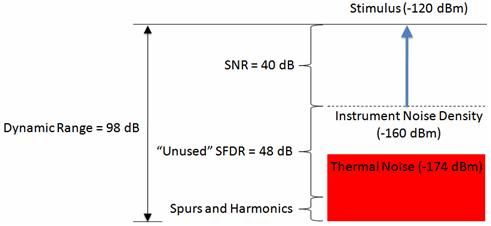Figure 2. Dynamic range lost due to instrument noise density

In the scenario where a DAC produces an output at +4 dBm, 124 dB of total attenuation is needed to produce an output at -120 dBm.  The PXIe-5672 vector signal generator addresses this requirement by applying 87 dB of IF/RF attenuation and 37 dB of digital attenuation, resulting in a total attenuation of 124 dB.

Based on the system described above, a combination of IF and RF attenuation can be applied by the instrument to lower the RF power level without introducing noise.  However, in some scenarios, adding additional attenuation with an external attenuator can provide added advantages.  The main two advantages, described in the subsequent sections, include improvements in amplitude accuracy and the extension of dynamic range at low power levels

### 3. Improving Amplitude Accuracy

While many factors affect amplitude accuracy, one of the biggest sources of amplitude error is VSWR, or voltage standing ratio.  In a perfect world, the impedance of both an RF instrument (typically 50 ohms) and the DUT (device under test) are matched.  However, even slight impedance mismatches produce VSWR, which cause the incident signal to be reflected back to the source.  Reflections can have a substantial affect on the test stimulus.  In fact, depending on the phase difference between the stimulus and reflection, these products can either increase or decrease the amplitude of the composite RF signal.  Typically VSWR is determined by measuring reflection coefficient of the materials being used.  This is noted in the equation below, where ‘ρ’ is the reflection coefficient: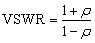Equation 6. VSWR as a function of reflection coefficient

Based on the VSWR of the transmission line, we can then calculate the return loss (RL) or amplitude of the reflection.  Return loss is determined by the following equation: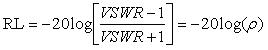Equation 7. Return loss as a function of VSWR

Note that while the amplitude of reflections are certainly predictable, the phase is not.  Because the reflection’s phase determines whether it will increase or decrease overall power, VSWR results in amplitude inaccuracy.

Fortunately, the effects of VSWR can be reduced substantially by using an external attenuator.  This is particularly helpful at lower power levels, where attenuation must already be used.  When using an attenuator, we can think of reflections of actually being attenuated twice.  This is illustrated in the figure below: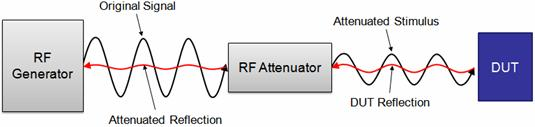Figure 3. Attenuator improves power accuracy by attenuating reflection

As figure 3 illustrates, the external attenuator attenuates the reflection caused by the DUT.  As a result, the affect of VSWR will be minimized and amplitude accuracy will be improved.

### 4. Dynamic Range Extension

A second motivation for using external attenuators to test low-sensitivity receivers is to reduce the noise density of the test signal.  In this scenario, the attenuator reduces both noise power and signal power.  As purely passive RF device, attenuators theoretically inject no noise into an RF signal.  For example, suppose we generate a 1 GHz tone at 0 dBm with a SNR of 80 dB.  In this scenario, the average noise density of the signal should be exactly -80 dBm/Hz.  If a 20 dB passive attenuator is applied to the signal, the SNR will theoretically remain constant.  Thus, the output of the attenuator will is -20 dBm and a noise power will is -100 dBm.  Thus, attenuators can be particularly helpful at power levels that are close to the average noise density of the RF instrument.  In this scenario, they can actually be used to extend our dynamic range by lowering the noise floor of the test stimulus.

For example, supposed we wanted to generate a signal with a SNR of 50 dB at a power level of -120 dBm.  Without attenuation, an example instrument (such as the PXIe-5672) will have an average noise density of -158 dBm/Hz.  Thus, our instrument can only provide us with 38 dB (-120 + 158) of dynamic range at this power level.  As a result an attenuator is required to attenuate the noise to the desired level of -170 dBm to produce an SNR of 50 dB.  In this scenario, the instrument is configured to have a power level of -100 dBm, and a 20 dB attenuator is used to attenuate the signal by 20 dB to -120 dBm.  Moreover, our noise level is attenuated to the theoretical minimum of -174 dBm.  Thus, our dynamic range has increased from 38 dB to 54 dB, which is the theoretical maximum dynamic range at the desired power level.  To illustrate this concept, we graphically represent the output power of a signal generator with and without attenuation.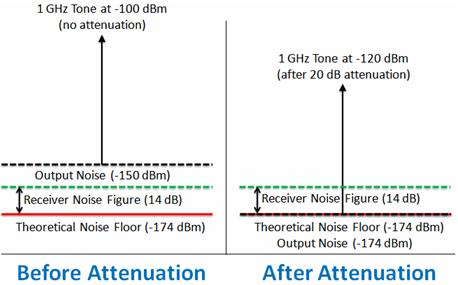Figure 4. Noise level before and after attenuation

As the figure above illustrates, an attenuator can be used to reduce both the output power and the output noise of a signal generator.  Note, however, that it is not able to attenuate a signal below the theoretical noise floor (thermal noise) at: -174 dBm/Hz.

Realistically, receiver sensitivity test will only require you to supply a noise figure that is only slightly below the noise figure of the receiver.  Thus, if the receiver has a noise figure of 14 dB, we should ensure that our test stimulus has an output noise density that is less than -160 dBm/Hz.  While the noise density of a generator can be reduced to -174 dBm/Hz, this will have diminishing benefits for test accuracy, since the noise introduced by receiver components will mask noise introduced by the generator.

As an example, we can use a PXI-5661 vector signal analyzer along with a PXI-5690 Pre-Amp to operate as a low-noise receiver.  Combined with the preamp, our VSA will should have a typical average noise density of -165 dBm/Hz.  This is equivalent to a high sensitivity receiver with a 9 dB noise figure.

First, we will use the PXI-5671 vector signal generate to generate a CW tone at 1 GHz.  At this power level the typical output noise density of the generator is specified at -158 dBm/Hz.  When the test stimulus is displayed in the vector signal analyzer, in 1 Hz bandwidth, we can observe that the displayed average noise floor is -157 dBm/Hz, shown below: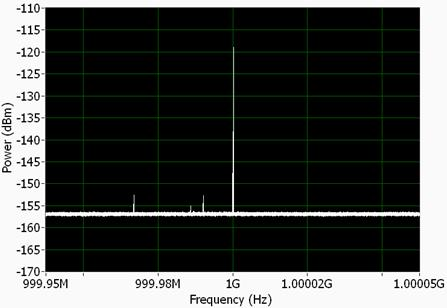Figure 5. Noise floor at -157 dBm/Hz without attenuation

As figure 5 illustrates, we are only using roughly 40 dB of the DAC’s dynamic range, since digital attenuation is being used.  To extend the dynamic range of our instrument, we simply add an external attenuator to our instrument.  With a 20 dB attenuator, our noise level should be reduced to the thermal noise level of -174 dBm/Hz. Note that the noise level in this case is attenuated by less than 20 dB, because it cannot be attenuated below the thermal noise level.  When applying attenuation, we must also adjust the output level to compensate for power lost.  Since our desired output power is -120 dBm, we configure the vector signal generator to have an output power of -100 dBm when using a 20 dB attenuator.

When viewing an attenuated output with our vector signal generator, the displayed average noise level (DANL) should be dominated by the noise figure of the analyzer, and not the generator.  With our PXI-5661/PXI-5690 VSA/Pre-amp combination, the typical noise floor is -165 dBm/Hz.  Our actual measurement is illustrated in the figure below: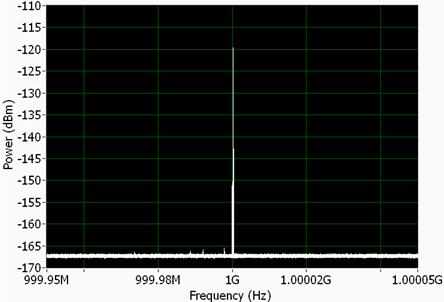Figure 6. Noise floor at -167 dBm/Hz without attenuation.

With attenuation added to the test stimulus, we can observe a noise density is -167 dBm/Hz.  As stated above, the noise density is dominated by the analyzer.  However, we can clearly see that the generator is outputting a signal with a noise level that is at least lower than -167 dBm/Hz.

While the example above seems fairly straightforward, it is important to clarify that noise can never be attenuated to lower than -174 dBm/Hz at room temperature.  In other words, while an attenuator is able to attenuate active noise that has been introduced by a component, it is NOT able to attenuate thermal noise at the theoretical noise floor.

The scenario above is often a source of confusion for RF engineers, because it is commonly known that the noise figure of any passive component is equal to its loss.  Noise figure (NF) and noise factor (F), are commonly described in terms of the ratio of input SNR to output SNR at the specific temperature of 290 K.  As described in the equations below, noise figure is simply the logarithmic equivalent of the noise factor measurement.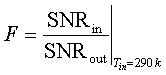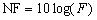Equations 8 and 9.  Relationship between noise figure (NF) and SNR

Based on the equations above, it might SEEM that use of an attenuator on the output of a vector signal generator would attenuate the signal strength without attenuating the noise power.  However, it is important to recognize the equation for noise figure ONLY relevant when the noise level is equal to the thermal noise density at -174 dBm/Hz.  In general, terms such as noise figure and noise factor should only be applied to wireless receivers, since the noise power from an antenna at 290K will be approximately -174 dBm/Hz.

For example, suppose a receiver observes a signal with a SNR of 60 dB at a power level of -114 dBm.  In this scenario, applying a 20 dB attenuator would attenuate the signal power by 20 dB to -134 dBm.  However, the noise level would remain unchanged, since the noise power is already at the thermal noise level.  Thus, when Pnoise = -174 dBm/Hz, the noise figure of a passive attenuator is equivalent to its loss.

On the other hand, output noise density from an RF generator is caused by active components and will result at levels that are sometimes significantly above the theoretical noise floor.  In this scenario, noise power can be attenuated to the level of the thermal noise floor.  As a result, high sensitivity receivers can be tested with a stimulus which has a noise power that is well below that of the signal generator.

### 5. Conclusion

When characterizing highly-sensitive receivers, it is important to test them with a realistic test stimulus.  While this produces the challenge of generating a signal with an extremely low noise density, it can be accomplished through the use of external attenuation.  In fact, as we have illustrated, external attenuator have the benefit of improving amplitude accuracy as well as extending the dynamic range of the instrument.

Customer Reviews
6 Reviews | Submit your review

wrong equation....  - Oct 10, 2016

Hi all, is there anyone realize that Equation 4 is incorrect? It is suppose to be "add" , not " minus" 30.... We are converting Watt into dBm, we add 30, not subtract 30. Correct me if I am wrong. Thanks.

Add attenuator to -174db/Hz  - Mar 31, 2016

Can someone please confirm if my understanding is correct: If I add 20 dB attenuator to in front of a hypothetical device with Noise power -174db/Hz then the output will still be -174db/Hz...correct ? However, If I add 20 dB attenuator to a device with noise power of -110 dbm, the output will be -130 dBm...correct ?

- Jun 8, 2010

good app note. but fig4 left side noise floor should be -158?

GPS Frequency Band Correction  - Apr 19, 2010

The 1575.42 MHz band is the L1 band, not the L2 band as indicated in the text. The typical signal level at the antenna is -130 dBm to -127 dBm for clear sky reception rather than -140 dBm. Older satellites were nearer the -130 dBm number, while newer satellites are closer to the -127 dBm number because of transmited power improvements.

- May 8, 2008

Isn't most of the Vector Signal Generator nowadays include a >100dB "external attenuators" inside the VSG itself?

Verizon guy  - Dec 10, 2007

Verizon guy is the one asking 'Can you hear me now' But that has changed too and the pitch is now 'It's the network.'

### Ratings

Rate this document